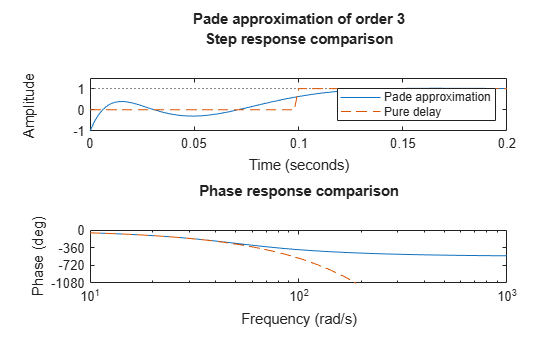Padé approximation of model with time delays

Description

pade approximates time delays by rational models. Such approximations are useful to model time delay effects such as transport and computation delays within the context of continuous-time systems. The Laplace transform of a time delay of T seconds is exp(–sT). This exponential transfer function is approximated by a rational transfer function using Padé approximation formulas .

[num,den] = pade(T,N) returns the Padé approximation of order N of the continuous-time I/O delay exp(–sT) in transfer function form. The row vectors num and den contain the numerator and denominator coefficients in descending powers of s. Both are Nth-order polynomials.

When invoked without output arguments, pade(T,N) plots the step and phase responses of the Nth-order Padé approximation and compares them with the exact responses of the model with I/O delay T. Note that the Padé approximation has unit gain at all frequencies.

sysx = pade(sys,N) produces a delay-free approximation sysx of the continuous delay system sys. All delays are replaced by their Nth-order Padé approximation. See Time Delays in Linear Systems for more information about models with time delays.

sysx = pade(sys,NU,NY,NINT) specifies independent approximation orders for each input, output, and I/O or internal delay. Here NU, NY, and NINT are integer arrays such that

• NU is the vector of approximation orders for the input channel

• NY is the vector of approximation orders for the output channel

• NINT is the approximation order for I/O delays (TF or ZPK models) or internal delays (state-space models)

You can use scalar values for NU, NY, or NINT to specify a uniform approximation order. You can also set some entries of NU, NY, or NINT to Inf to prevent approximation of the corresponding delays.

Examples

collapse all

Compute a third-order Padé approximation of a 0.1-second I/O delay.

s = tf('s');
sys = exp(-0.1*s);
sysx =

-s^3 + 120 s^2 - 6000 s + 1.2e05
--------------------------------
s^3 + 120 s^2 + 6000 s + 1.2e05

Continuous-time transfer function.

Here, sys is a dynamic system representation of the exact time delay of 0.l s. sysx is a transfer function that approximates that delay.

Compare the time and frequency responses of the true delay and its approximation. Calling the pade command without output arguments generates the comparison plots. In this case the first argument to pade is just the magnitude of the exact time delay, rather than a dynamic system representing the time delay.Limitations

High-order Padé approximations produce transfer functions with clustered poles. Because such pole configurations tend to be very sensitive to perturbations, Padé approximations with order N>10 should be avoided.

References

 Golub, G. H. and C. F. Van Loan, Matrix Computations, Johns Hopkins University Press, Baltimore, 1989, pp. 557-558.